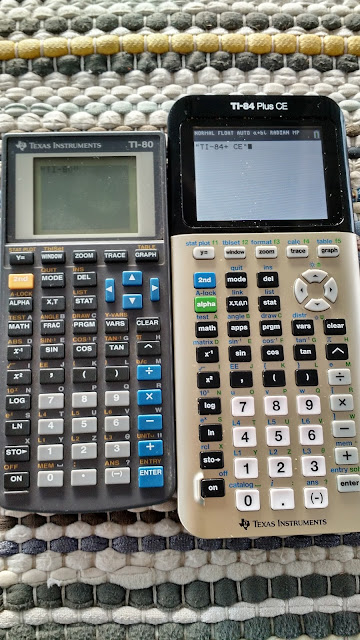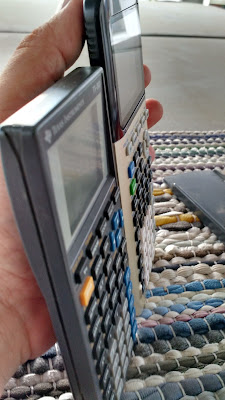## Monday, September 4, 2017

### Retro Review: Texas Instruments TI-80TI-80TI-80 (left), TI-84 Plus CE (right)Look how thin the TI-80 is
Retro Review: Texas Instruments TI-80

First, thank you Nano for the TI-80 (along with giving me a pair of slide rules and an astronomy poster)!  Much appreciated!

Essentials

Company:  Texas Instruments
Years:  1995
Type:  Graphing, Programming
Memory:  7,034 bytes
Operating System: Algebraic
Memory Registers: 27 (A-Z, θ)
Screen:  Monochrome

Batteries:  2 CR2032 batteries

Graphing Modes:  Function (4), Parametric (3).  Table included.

Regressions:  6: Linear (ax + b), Quadratic, Linear (a + bx), Logarithmic, Exponential, Power

Lists: Up to 99 entries per lists, 6 lists available (L1 through L6)

Matrices: none

Complex Numbers:  none

Keyboard

The keyboard is what one would expect on a Texas Instruments graphing calculator: nice and responsive.

Screen

The screen is small.  Not kidding.  The screen is only 48 x 64 pixels big, accompanying 8 lines of 16 characters.  That means that the font is small.  What is wild is that the pi symbol (π) does not conform to the rest of the font, and is twice as long as the rest of the characters.

The screen is still bigger than the mini-graphing calculators such as the Casio fx-6300g or the Hewlett Packard HP-9g.

Is the TI-80 a simplified TI-81?

For the most part, no.  Sure, the TI-80 does not have matrices and hyperbolic functions (sinh, cosh, etc) like the TI-81.  However, the TI-80 has fractions (see the next section), integer division and remainder function, random integer, a complementary table mode, and lists.  The number of stat plots increased to 3, which they don’t have to depend on the statistics mode.

As far as programming memory, the TI-80 beats the TI-81: 7,034 bytes to 2,400 bytes.  Also, you can go beyond 37 programs for the TI-80, as the names are not restricted to one character.

Fractions

The TI-80 has a dedicated fraction menu, which allow users to convert between improper and proper form, as well as conversion between fraction and decimal approximation.  The Manual Simplification mode allows fractions to not be automatically simplified on calculation.

To enter fractions, the format is:  A _ B / C
Note that the slash is bold.  Merely pressing the division key will not register the fraction.

To separate the whole part from the fraction, press [ 2nd ] [ + ] (UNIT_).

To separate the numerator from the denominator, press [ 2nd ] [ ÷ ] (b/c).

Example:  Enter 2 3/4
Keystrokes:  2 [ 2nd ] [ + ] 3 [ 2nd ] [ ÷ ] 4

According to Datamath (http://www.datamath.org/Graphing/TI-80.htm ), the TI-80 would get replaced with the TI-73 in 1998.  This may mean that the TI-80 became the base for the TI-73 series (TI-73, TI-73 explorer).

Lists

The TI-80 allows for 6 lists, each with a 99 element capacity.  Arithmetic can be operated on two same-sized lists, on an element-by-element functions.  Lists functions include sorting, dimension, minimum, maximum, sum of the elements, product of the elements, and sequence generation.

Programming

Programming is fairly basic for the TI-81.  Commands:
If-Then-Else-End Structure (IF, THEN, ELSE, END)
Quick if structure
For-End structure (no IS>, DS< this time) (FOR, END)
Labels:  one character and local labels (LBL, GOTO)
Subroutines (PRGM_, RETURN)
Drawing commands include points, shading (three types, general, Y<, Y>)

Since the only built-in calculus function of the TI-80 is numerical derivation (NDERIV), two programs for Newton’s Method and Simpson’s Rule are presented below.

TI-80 Program:  SOLVEY1  (Newton’s Method)

80 bytes
The equation is stored in Y1.  The program solves for X in Y1(X) = 0

INPUT “GUESS:”, X
LBL 0
X-Y1/NDERIV(Y1,X,X)→N
IF ABS (X-N)>1E-10
THEN
N→X
GOTO 0
END
N→X
DISP “X = “, X

Example: X^2-3X+1, guess X = 3
Result:  X = 2.618033989

TI-80 Program: SIMPY1 (Integral, Simpson’s Rule)

140 bytes
The equation is stored in Y1.  The program calculates ∫(Y1,X,A,B)

INPUT “A:”,A
INPUT “B:”,B
INPUT “N (EVEN):”,N
(B-A)/N→H
0→T
FOR(I,1,N-1)
A+IH→X
T+2*Y1→T
IF FPART(I/2)≠0
2*Y1+T→T
END
(T+Y1(A)+Y1(B))H/3→T
DISP “INTEGRAL:”,T

Example: X^2-3X+1, with A = 0 to B = 5 and N = 10
Result:  X = 9.166666667

Final Verdict

The TI-80 is a nice introductory calculator, and thanks to programming a lot can be done with it.  I wish the screen was bigger and degree/degrees-minutes-seconds conversions were available, but other than that, it was a great calculator which provides a lot of features (maybe not as intimidating as more advanced calculators).

It is a nice calculator to add to the collection, and I thank you Nano immensely.

Eddie

This blog is property of Edward Shore, 2017.